Definitions of Square Dance Calls and Concepts

Index -->  Plus  |  A1  |  A2  |  C1  |  C2  |  C3A  |  C3B  |  C4  |  NOL  |
Definitions (Text Only) -->  Plus  |  A1  |  A2  |  C1  |  C2  |  C3A  |  C3B  |  C4  |  NOL  |
 Find call:

 \$B8@8l(B

From Columns. EN: 10
Column \$B\$+\$i(B. JP: 10

The First n dancers Peel Off to end in a Line of n, Step Ahead as necessary to form a Concentric Line of 2n and Bend The Line of 2n as the other dancers Extend (using Circulates) to form a compact formation, Trade, and Roll. EN: 20
\$B;O\$a\$N(B n \$B?M\$,(B Peel Off \$B\$r\$7(B n \$B?M\$N(B Line \$B\$H\$J\$j(B, \$BI,MW\$J\$iA0?J\$7(B Concentric \$B\$J(B 2 \$B\$D\$N(B n \$B?M\$N(B Line \$B\$r:n\$j(B, 2 \$B\$D\$N(B n \$B?M\$G(B Bend The Line \$B\$r\$7\$^\$9(B. \$BB>\$N?M\$O(B Extend (Circulate \$B\$r;H\$\$(B) \$B\$K\$h\$j%3%s%Q%/%H\$J(B formation \$B\$r:n\$j(B, Trade \$B\$r\$7(B, Roll \$B\$r\$7\$^\$9(B. JP: 20

n Step(s) At A Time can be fractionalized into thirds. EN: 30
n Step(s) At A Time \$B\$O(B 3 \$B\$D\$N%Q!<%H\$N%3!<%k\$G\$9(B. JP: 30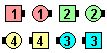\$B\$B;O\$a\$N(B formation: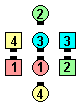1 Step At A Time\$B\$N8e(B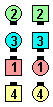2 Steps At A Time\$B\$N8e(B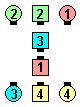3 Steps At A Time\$B\$N8e(B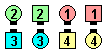4 Steps At A Time\$B\$N8e(B

2 Steps At A Time: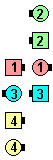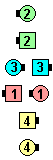2 Steps At A Time\$B\$NA0(B First 2 Peel Off as Last 2 Circulate(1/3)\$B\$N8e(B Outsides Step Ahead as Centers Trade(2/3)\$B\$N8e(B Outsides Bend The Line as Centers Roll\$B\$N8e(B (\$B=*\$o\$j(B)

\$B1~MQ(B:
• Magic (Column) 1, 2, 3 Step(s) At A Time.
• From Columns of 3. EN: 40
3 \$B?M\$N(B Column \$B\$+\$i(B. JP: 40
• From Completed Double Pass Thru: 2 Steps At A Time 2/3: Peel Off and Touch to end in a R-H Tidal Wave. EN: 50
Completed Double Pass Thru \$B\$+\$i(B: 2 Steps At A Time 2/3: Peel Off \$B\$r\$7\$F(B Touch \$B\$r9T\$\$(B \$B1&JP: 50

Cross n Step(s) At A Time [C3B]:
First n dancers Trail Off instead of Peel Off. EN: 818
\$B;O\$a\$N(B n \$B?M\$O(B Peel Off \$B\$NBe\$o\$j\$K(B Trail Off \$B\$r9T\$\$\$^\$9(B. JP: 818\$B\$B;O\$a\$N(B formation: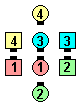Cross 1 Step At A Time\$B\$N8e(B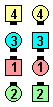Cross 2 Steps At A Time\$B\$N8e(B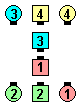Cross 3 Steps At A Time\$B\$N8e(B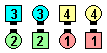Cross 4 Steps At A Time\$B\$N8e(B

Criss Cross n Step(s) At A Time [C4]:
First n dancers Trail Off, Step Ahead, and (Concentric) Bend The Line of 2n as others Extend to form a compact formation & Slither, Trade, and Roll. EN: 819
\$B;O\$a\$N(B n \$B?M\$O(B Trail Off \$B\$r\$7(B, Step Ahead \$B\$r\$7(B, 2 \$B\$D\$N(B n \$B?M\$G(B (Concentric) Bend The Line \$B\$r\$7\$^\$9(B. \$BB>\$N?M\$O(B Extend \$B\$K\$h\$j%3%s%Q%/%H\$J(B formation \$B\$r:n\$j(B; Slither, Trade, Roll \$B\$r\$7\$^\$9(B. JP: 819Choreography for n Step(s) At A TimeComments? Questions? Suggestions?

https://www.ceder.net/def/stepsatatime.php?level=master&language=japan&action=edit
03-August-2020 17:13:00